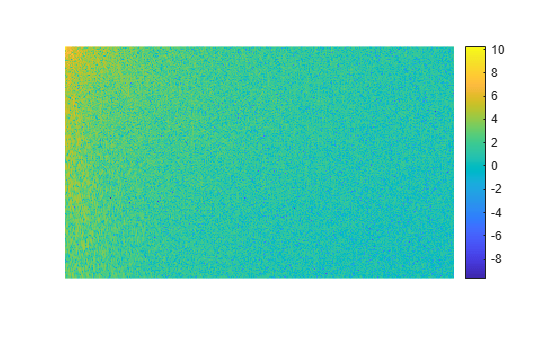# dct2

## 语法

``B = dct2(A)``
``B = dct2(A,m,n)``
``B = dct2(A,[m n])``

## 说明

``B = dct2(A)` 返回 `A` 的二维离散余弦变换。矩阵 `B` 包含离散余弦变换系数 B(k1,k2)。`
``B = dct2(A,m,n)` 和在应用变换之前，`B = dct2(A,[m n])` 用 `0` 对矩阵 `A` 进行填充，使其大小为 `m`×`n`。如果 `m` 或 `n` 小于 `A` 的对应维度，则 `dct2` 在变换前对 `A` 进行裁切。`

## 示例

```RGB = imread('autumn.tif'); I = im2gray(RGB);```

`J = dct2(I);`

```imshow(log(abs(J)),[]) colormap parula colorbar````J(abs(J) < 10) = 0;`

```K = idct2(J); K = rescale(K);```

```montage({I,K}) title('Original Grayscale Image (Left) and Processed Image (Right)');```## 详细信息

### 离散余弦变换

MN 分别是 `A` 的行和列大小。

## 提示

• 如果将 DCT 应用于实数数据，结果也是实数。DCT 倾向于集中信息，这在图像压缩应用中很有用。

• 要进行 DCT 逆变换，请使用 `idct2`

 Jain, Anil K., Fundamentals of Digital Image Processing, Englewood Cliffs, NJ, Prentice Hall, 1989, pp. 150–153.

 Pennebaker, William B., and Joan L. Mitchell, JPEG: Still Image Data Compression Standard, Van Nostrand Reinhold, 1993.